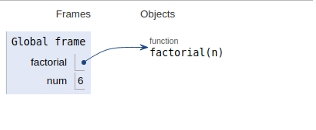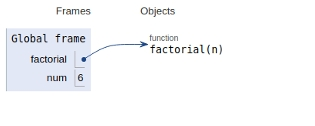# Python Program for factorial of a number

In this article, we will learn about the solution and approach to solve the given problem statement.

Problem statement −Our task to compute the factorial of n.

Factorial of a non-negative number is given by −

n! = n*n-1*n-2*n-3*n-4*.................*3*2*1

We have two possible solutions to the problem

• Recursive approach
• Iterative approach

## Example

Live Demo

def factorial(n): # recursive solution
if (n==1 or n==0):
return 1
else:
return n * factorial(n - 1)
# main
num = 6
print("Factorial of",num,"is", factorial(num))

## Output

('Factorial of', 6, 'is', 720)

All the variables are declared in global scope as shown in the image belowApproach 2 −Iterative Approach

## Example

Live Demo

def factorial(n):# iterative solution
fact=1
for i in range(2,n+1):
fact=fact*i
return fact
# main
num = 6
print("Factorial of",num,"is", factorial(num))

## Output

('Factorial of', 6, 'is', 720)

All the variables are declared in global scope as shown in the image below